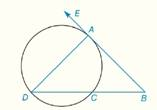Chapter 6.CR, Problem 9CRElementary Geometry For College St...

7th Edition
Alexander + 2 others
ISBN: 9781337614085

Solutions

Chapter
SectionElementary Geometry For College St...

7th Edition
Alexander + 2 others
ISBN: 9781337614085
Textbook Problem

In Review Exercises 5 to 10, B A → is tangent to the circle at point A in the figure shown. G i v e n :           C   i s   t h e   m i d p o i n t   o f   A C D ^   a n d     m ∠ B = 40 °       F i n d :             m A D ^ ,   m A C ^ , m D C ^To determine

To find: mAD^,mAC^, mDC^

Explanation

Theorem:

1) The measure of an angle formed when two secants intersect at a point outside the circle is one-half the difference of the measures of two intercepted arcs.

2) The measure of an inscribed angle of a circle is one-half the measure of its intercepted arc.

Calculation:

Given that

mB=40° and C is the midpoint of mACD^ and BA is tangent to the circle at point A.

By theorem, the measure of an angle (mB) formed when two secants (BD and BA) intersect at a point (B) outside the circle is one-half the difference of the measures of two intercepted arcs AD^ and AC^ .

Since, C is the midpoint of mACD^, mAC^=mDC^

Still sussing out bartleby?

Check out a sample textbook solution.

See a sample solution

The Solution to Your Study Problems

Bartleby provides explanations to thousands of textbook problems written by our experts, many with advanced degrees!

Get Started

Change each decimal to a percent: 1.2

Elementary Technical Mathematics

In Exercises 4756, solve the given equation for the indicated variable. 27=32x1

Finite Mathematics and Applied Calculus (MindTap Course List)

Expand sin(+45) and then simplify.

Trigonometry (MindTap Course List)

Evaluate the integral. 30. 0/4tan4tdt

Single Variable Calculus: Early Transcendentals

Which is the best graph of r = 1 − sin θ for 0 ≤ θ ≤ π?

Study Guide for Stewart's Multivariable Calculus, 8th